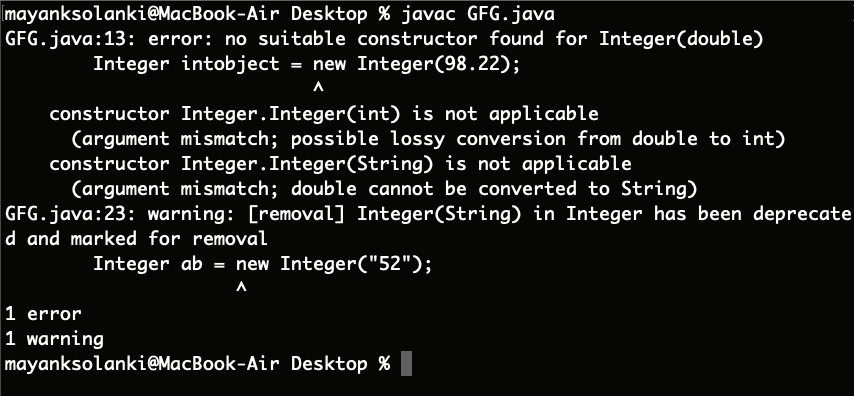# Integer intValue() Method in Java

• Difficulty Level : Basic
• Last Updated : 03 May, 2022

intValue() of Integer class that is present inside java.lang package is an inbuilt method in java that returns the value of this integer as an int which is inherited from Number Class. The package view is as follows:

```--> java.lang Package
--> Integer Class
--> intValue() Method    ```

Syntax:

`public int intValue()`

Return Type: A numeric value that is represented by the object after conversion to the integer type.

Note: This method is applicable from java version 1.2 and onwards.

Now we will be covering different numbers such as positive, negative, decimal, and even strings.

• For a positive integer
• For a negative number
• For a decimal value and string

Case 1: For a positive integer

Example:

## Java

 `// Java Program to Illustrate``// the Usage of intValue() method``// of Integer class` `// Importing required class/es``import` `java.lang.*;` `// Main class``public` `class` `GFG {` `    ``// Main driver method``    ``public` `static` `void` `main(String[] args)``    ``{` `        ``// Creating object of Integer class inside main()``        ``Integer intobject = ``new` `Integer(``68``);` `        ``// Returns the value of this Integer as an int``        ``int` `i = intobject.intValue();` `        ``// Printing the value above stored in integer``        ``System.out.println(``"The integer Value of i = "` `+ i);``    ``}``}`

Output:

`The integer Value of i = 68`

Case 2:  For a negative number

Example:

## Java

 `// Java program to illustrate the``// use of intValue() method of``// Integer class of java.lang package` `// Importing required classes``import` `java.lang.*;` `// Main class``public` `class` `GFG {` `    ``// Main driver method``    ``public` `static` `void` `main(String[] args)``    ``{` `        ``// Creating an object of Integer class``        ``Integer intobject = ``new` `Integer(-``76``);` `        ``// Returns the value of this Integer as an int``        ``int` `i = intobject.intValue();` `        ``// Printing the integer value above stored on``        ``// console``        ``System.out.println(``"The integer Value of i = "` `+ i);``    ``}``}`

Output:

`The integer Value of i = -76`

Case 3: For a decimal value and string.

Example:

## Java

 `// Java Program to illustrate``// Usage of intValue() method``// of Integer class of java.lang package` `// Importing required classes``import` `java.lang.*;` `// Main class``class` `GFG {` `    ``// Main driver method``    ``public` `static` `void` `main(String[] args)``    ``{``        ``// Creating an object of Integer class``        ``Integer intobject = ``new` `Integer(``98.22``);` `        ``// Using intValue() method``        ``int` `i = intobject.intValue();` `        ``// Printing the value stored in above integer``        ``// variable``        ``System.out.println(``"The integer Value of i = "` `+ i);` `        ``// Creating another object of Integer class``        ``Integer ab = ``new` `Integer(``"52"``);` `        ``int` `a = ab.intValue();` `        ``// This time printing the value stored in "ab"``        ``System.out.println(``"The integer Value of ab = "``                           ``+ a);``    ``}``}`

Output:Note: It returns an error message when a decimal value is given. For a string this works fine.

My Personal Notes arrow_drop_up